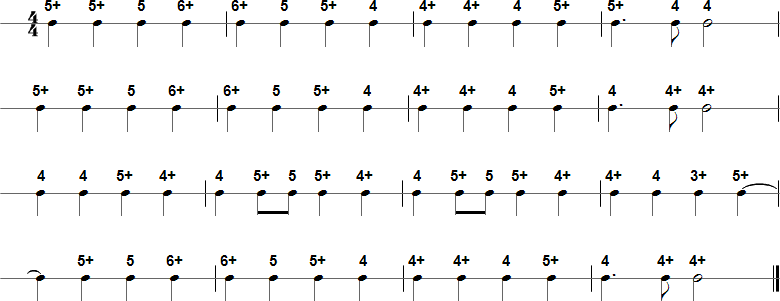Browse by Title:

# Ode to Joy - Harmonica Tablature and Sheet Music## Details

• Title(s): Ode to Joy
• Composer: Ludwig van Beethoven
• Category: Classical

## Tab in Text Format

5+ 5+ 5 6+ 6+ 5 5+ 4 4+ 4+ 4 5+ 5+ 4 4 5+ 5+ 5 6+ 6+ 5 5+ 4 4+ 4+ 4 5+ 4 4+ 4+ 4 4 5+ 4+ 4 5+ 5 5+ 4+ 4 5+ 5 5+ 4+ 4+ 4 3+ 5+ 5+ 5 6+ 6+ 5 5+ 4 4+ 4+ 4 5+ 4 4+ 4+